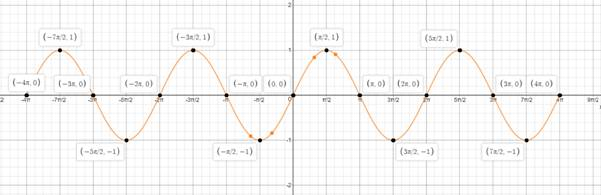# Graph each of the following between x = − 4 π and x = 4 π . y = sin xFindFindarrow_forward

### Bundle: Trigonometry, Loose-leaf V...

8th Edition
Charles P. McKeague + 1 other
Publisher: Cengage Learning
ISBN: 9781337131063

#### Solutions

Chapter
SectionFindFindarrow_forward

### Bundle: Trigonometry, Loose-leaf V...

8th Edition
Charles P. McKeague + 1 other
Publisher: Cengage Learning
ISBN: 9781337131063
Chapter 4, Problem 1CT
Textbook Problem
43 views

## Graph each of the following between x = − 4 π and x = 4 π . y = sin x

To determine

To graph:

The equation y=sinx,4πx4π

### Explanation of Solution

Given information:

The equation y=sinx,4πx4π

Calculation:

The amplitude is 1.

The coefficient of x, B = 1.

Therefore, the period of the function = 2πB=2π1=2π

The period of the function is 2π.

Divide the period 2π by 4, we get π2. To mark x-axis, we begin at x = 4π and add increments of π2.

Graph:

The graph of the equation y=sinx,4πx4π :#### The Solution to Your Study Problems

Bartleby provides explanations to thousands of textbook problems written by our experts, many with advanced degrees!

Get Started

Find more solutions based on key concepts
In Exercises 29-34, find an equation of the circle that satisfies the given conditions. 33. Center (2, 3) and p...

Applied Calculus for the Managerial, Life, and Social Sciences: A Brief Approach

Graphical Addition Use graphical addition to sketch the graph of f + g. 22.

Precalculus: Mathematics for Calculus (Standalone Book)

Write the sum in sigma notation. 12. 3+4+5+6+7

Single Variable Calculus: Early Transcendentals

True or False: f(x) = x2 is decreasing for 10 x 1.

Study Guide for Stewart's Single Variable Calculus: Early Transcendentals, 8th

Explain how the purpose of a correctional study differs from the purpose of an experimental study.

Research Methods for the Behavioral Sciences (MindTap Course List)

The following 20 observations are for two quantitative variables, x and y. Develop a scatter diagram for the r...

Modern Business Statistics with Microsoft Office Excel (with XLSTAT Education Edition Printed Access Card) (MindTap Course List)

Round all answers to two decimal places. Using Exponential modelsIn Exercises S-15 through S-24, we use exponen...

Functions and Change: A Modeling Approach to College Algebra (MindTap Course List)

In Problems 1320 use (20) to find the general solution of the given differential equation on (0, ). 13. xy + 2y...

A First Course in Differential Equations with Modeling Applications (MindTap Course List)